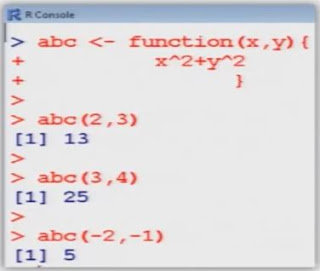# Basic of Calculations _Functions_Matrices in R Language

Function :-

Function are a bunch of commands grouped together in a sensible unit.

Functions take input arguments, do calculations (or make some graphics, call other functions) and produce some output and return a result in a variable. The returned variable can be a complex construct, like a list.

Syntax

Name <- function(Argument1, Argument2, ...)
{
expression
}
Where expression is a single command or a group of commands
• Function arguments can be given a meaningful name
• Function arguments can be set to default values
• Functions can have the special argument '...'
Functions (Single variable)

The sign <- is furthermore used for defining functions:
> abc <- function(x) {
x^2
}
> abc (3)
  9

>abc (6)
  36

> abc (-2)
   4

Function (Two variables)>abc  <- function (x,y) {
x^2+y^2
}
> abc (2,3)
  13
> abc (3,4)
  25
> abc  (-2,-1)
  5

Matrix
• Matrices are important objects in any calculation.
• A matrix is a rectangular array with p rows and n columns.
• An element in the i-th row and j-th column is denoted by xij (book version) or x[i,j] ("program version"), i = 1,2,.....,n, j = 1,2,...,p.
• An element of a matrix can also be an object, for example a string. However, in mathematics, we are mostly interested in numerical matrices, whose element are generally real numbers
In R, a 4⤫2-matrix x can be created with a following command:

>x <- matrix (nrow = 4 , ncol = 2, data = c(1,2,3,4,5,6,7,8) )

We see:
• The parameter nrow defines the row number of a matrix.
• The parameter ncol defines the column number of a matrix.
• The parameter data assigns specified values to the matrix element.
• The value from the parameters are written column-wise in matrix.

>  x
[,1]          [,2]
[1,]           1             5
[2,]           2             6
[3,]           3             7
[4,]           4             8
• One can access a single element of a matrix with x[i,j] :
> x [3,2]
   7